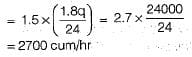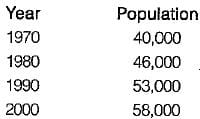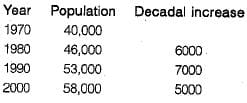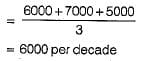Test: Water Demand

# Test: Water Demand

Test Description

## 10 Questions MCQ Test GATE Civil Engineering (CE) 2023 Mock Test Series | Test: Water Demand

Test: Water Demand for Civil Engineering (CE) 2023 is part of GATE Civil Engineering (CE) 2023 Mock Test Series preparation. The Test: Water Demand questions and answers have been prepared according to the Civil Engineering (CE) exam syllabus.The Test: Water Demand MCQs are made for Civil Engineering (CE) 2023 Exam. Find important definitions, questions, notes, meanings, examples, exercises, MCQs and online tests for Test: Water Demand below.
Solutions of Test: Water Demand questions in English are available as part of our GATE Civil Engineering (CE) 2023 Mock Test Series for Civil Engineering (CE) & Test: Water Demand solutions in Hindi for GATE Civil Engineering (CE) 2023 Mock Test Series course. Download more important topics, notes, lectures and mock test series for Civil Engineering (CE) Exam by signing up for free. Attempt Test: Water Demand | 10 questions in 30 minutes | Mock test for Civil Engineering (CE) preparation | Free important questions MCQ to study GATE Civil Engineering (CE) 2023 Mock Test Series for Civil Engineering (CE) Exam | Download free PDF with solutions
 1 Crore+ students have signed up on EduRev. Have you?
Test: Water Demand - Question 1

### Which one of the following practices, causes reduction in the per capita water consumption?

Detailed Solution for Test: Water Demand - Question 1

Good Quality water, hotter climate and modern living, all these factors will increase the per capita water consumption.
However, metering system will reduce the consumption as people will be cautious while using the water as they need to pay for it.

Test: Water Demand - Question 2

### The multiplying factor, as applied to obtain the maximum daily water demand, in relation to the average i.e. per capita daily demand, is

Detailed Solution for Test: Water Demand - Question 2

Maximum daily demand
= 1.8 x Average daily demand Maximum hourly demand
= 1.5 x Maximum daily demand

Test: Water Demand - Question 3

### If the average daily water consumption of a city is 24000 cum, the peak hourly demand (of the maximum day) will be

Detailed Solution for Test: Water Demand - Question 3

Maximum hourly consumption of the maximum day i.e. peak demandTest: Water Demand - Question 4

The total water requirement of a city is generally assessed on the basis of

Detailed Solution for Test: Water Demand - Question 4

For general community purposes, the total draft is not taken as the sum of maximum hourly demand and fire demand, but is taken as the sum of maximum daily demand and fire demand, or the maximum hourly demand, whichever is more. The maximum daily demand (i.e. 1.8 times the average daily demand) when added to fire draft,for working out total draft, is known as coincident draft.

Test: Water Demand - Question 5

As compared to the geometrical increase method of forecasting population, the arithmetical increase method gives.

Detailed Solution for Test: Water Demand - Question 5

In geometric increase method compounding is done every decade unlike in arithmetic increase method. Thus geometrical increase method gives higher value than the arithmetic increase method.

Test: Water Demand - Question 6

The growth of population can be conveniently represented by a curve, which is amenable to mathematical solution, The type of this curve is

Detailed Solution for Test: Water Demand - Question 6

Equation of logistic curve is given by,
In [(Ps - P)/(Ps - P0)] = -kPst
where,
P0 = The population at the start pt. of the curve A
Ps = Saturation population
P = Population at any time t from the origin A
K = Constant

Test: Water Demand - Question 7

The average domestic water consumption per capita per day for an Indian city, as per IS 1172- 1963, may be taken as

Test: Water Demand - Question 8

The total water consumption per capita per day, including domestic, commercial, and industrial demands, for an average Indian city, as per IS Code, may be taken as

Detailed Solution for Test: Water Demand - Question 8

Break up of per capita demand (q) for an average Indian cityTest: Water Demand - Question 9

The population figures in a growing town are as follows:The predicted population in 2010 by Arithmetic Regression method is

Detailed Solution for Test: Water Demand - Question 9∴ Design growth rate,In 2010 population will be,
P = 58000 + 6000 = 64000

Test: Water Demand - Question 10

The pipe mains carrying water from the source to the reservoir are designed for the

Detailed Solution for Test: Water Demand - Question 10

The pipe mains carrying water from the source to the reservoir are designed for the maximum daily draft = 1.8 times the average daily draft.
However, pipes for distributing water to households etc. are designed for maximum hourly draft of the maximum day = 2.7 times the average daily draft.

## GATE Civil Engineering (CE) 2023 Mock Test Series

26 docs|292 tests
 Use Code STAYHOME200 and get INR 200 additional OFF Use Coupon Code
Information about Test: Water Demand Page
In this test you can find the Exam questions for Test: Water Demand solved & explained in the simplest way possible. Besides giving Questions and answers for Test: Water Demand, EduRev gives you an ample number of Online tests for practice

## GATE Civil Engineering (CE) 2023 Mock Test Series

26 docs|292 tests